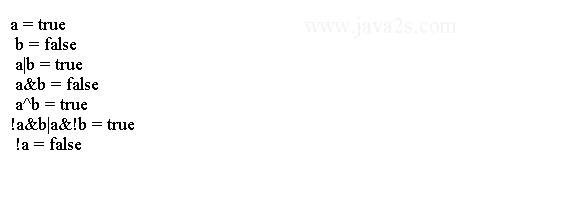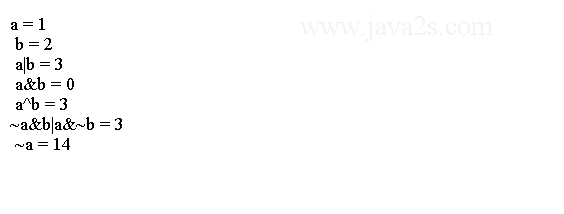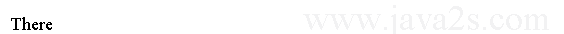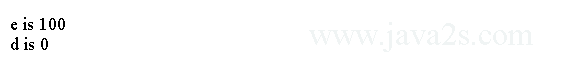# Java Tutorial - Java Boolean Operator

The Boolean logical operators operate on boolean operands.

## Logical Operator List

The following table lists all Java boolean logical operators.

Operator Result
& Logical AND
| Logical OR
^ Logical XOR (exclusive OR)
|| Short-circuit OR
&& Short-circuit AND
! Logical unary NOT
&=AND assignment
|= OR assignment
^= XOR assignment
== Equal to
!= Not equal to
? : Ternary if-then-else

## True table

The following table shows the effect of each logical operation:

A B A | B A & BA ^ B!A
FalseFalseFalseFalse FalseTrue
True FalseTrue False True False
FalseTrue True False True True
True True True True FalseFalse

The following program demonstrates the boolean logical operators.

```
public class Main {
public static void main(String args[]) {
boolean a = true;
boolean b = false;
boolean c = a | b;
boolean d = a & b;
boolean e = a ^ b;
boolean f = (!a & b) | (a & !b);
boolean g = !a;
System.out.println(" a = " + a);
System.out.println(" b = " + b);
System.out.println(" a|b = " + c);
System.out.println(" a&b = " + d);
System.out.println(" a^b = " + e);
System.out.println("!a&b|a&!b = " + f);
System.out.println(" !a = " + g);
//  www  .  j  a v  a2  s.c om
}
}
]]>
```

The output:## Example

The following program demonstrates the bitwise logical operators:

```
public class Main {
public static void main(String args[]) {
int a = 1;//from  w w  w . j av  a2 s. c o  m
int b = 2;
int c = a | b;
int d = a & b;
int e = a ^ b;
int f = (~a & b) | (a & ~b);
int g = ~a & 0x0f;

System.out.println(" a = " + a);
System.out.println(" b = " + b);
System.out.println(" a|b = " + c);
System.out.println(" a&b = " + d);
System.out.println(" a^b = " + e);
System.out.println("~a&b|a&~b = " + f);
System.out.println(" ~a = " + g);

}
}
```

Here is the output from this program:## Java Logical Operators Shortcut

The `OR` operator results in true when one operand is `true`, no matter what the second operand is. The `AND` operator results in false when one operand is `false`, no matter what the second operand is. If you use the `||` and `&&`, Java will not evaluate the right-hand operand when the outcome can be determined by the left operand alone.

The following code shows how you can use short-circuit logical operator to ensure that a division operation will be valid before evaluating it:

```
public class Main {
public static void main(String[] argv) {
int denom = 0;
int num = 3;// ww  w .  j  a v  a  2s  .  c  om
if (denom != 0 && num / denom > 10) {
System.out.println("Here");
} else {
System.out.println("There");
}
}
}
```

The output:If we want to turn of the shortcut behaviour of logical operators we can use & and |.

## Example 2

The following code uses a single & ensures that the increment operation will be applied to `e` whether `c` is equal to 1 or not.

```
public class Main {
public static void main(String[] argv) {
int c = 0;/*  www  .j  a  va2 s. c om*/
int e = 99;
int d = 0;
if (c == 1 & e++ < 100)
d = 100;

System.out.println("e is " + e);
System.out.println("d is " + d);
}
}
```

The output: